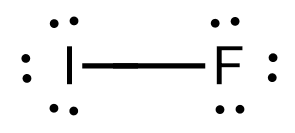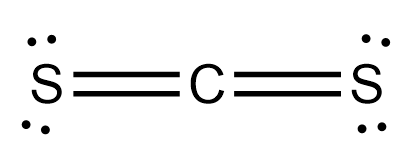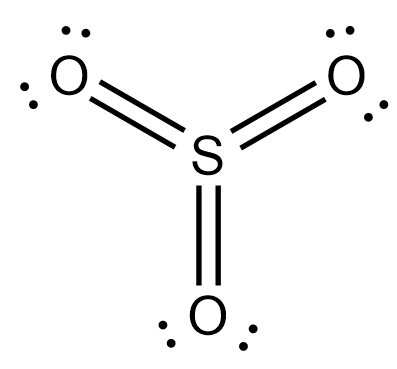# Problem: Predict whether each of the following molecules is polar or non-polar:IF, CS2, SO3, PCl3, SF6, IF5

###### FREE Expert Solution

IF, CS2, SO3, PCl3, SF6, IF5

a. For IF

(1) No central atom

(2) I → Group 7A → 7 valence electrons

F → Group 7A → 7 valence electrons

(3) Lewis structure will appear as:F is more electronegative than I; Hence a dipole moment exists

Therefore, IF is polar

b. For CS2

(1) C → less EN than S → C is central atom

(2) C → Group 4A → 7 valence electrons

S → Group 6A → 6 valence electrons

(3) Lewis structure will appear as:Structure appears to be linear (AX2) where each C=S cancels each other's dipole

Therefore, CS2 is nonpolar

c. For SO3

(1) S → less EN than O → S is central atom

(2) S → Group 6A → 6 valence electrons

O → Group 6A → 6 valence electrons

(3) Lewis structure will appear as:Structure appears to be trigonal planar (AX3) where each S=O cancels each other's dipole

Therefore, SO3 is nonpolar###### Problem Details

Predict whether each of the following molecules is polar or non-polar:

IF, CS2, SO3, PCl3, SF6, IF5